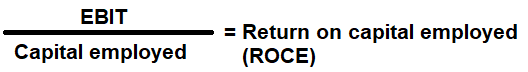# Return on Capital Employed Ratio Calculator

Key in the Net Income Values from your Income Statement. Total Assets and Current Liabilities Values from your Balance Sheet to the respective fields given below and then click Calculate to get the desired result.

# Return on Assets Ratio Calculator - Glossary:

Return on Capital Employed (ROCE): Shows the proportion of EBIT to capital employed. In other words, return on capital employed shows investors how many dollars in profits each dollar of capital employed generates.

### Formula:### How to use this equation?

The values for net operating profit or earnings before interest and tax (EBIT) can be obtained from income statement and to calculate capital employed - obtain the values of total assets and current liabilities from the balance sheet. To use this ratio, divide earnings before interest and tax (EBIT) by the capital employed.

Earnings before interest and tax (EBIT):
Net income or profit before deducting all its interest & taxes.

Capital Employed:
It is the total amount of equity invested in a company. It is the sum of shareholders' equity and current liabilities. It can be simplified as total assets minus current liabilities.

Example:
Return on capital employed ratio for a company with a reported EBIT of \$100,000; \$200,000 of total assets and \$50,000 of current liabilities is 0.6666 or 66.66%. It means that, a company yields a return of \$0.66 per dollar for its capital employed.